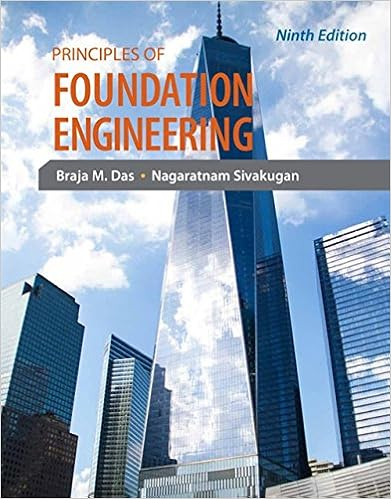# Required compute the following quantities you should

• Notes
• dominiquefierro1012
• 5
• 100% (14) 14 out of 14 people found this document helpful

This preview shows page 3 - 4 out of 5 pages.

##### We have textbook solutions for you!
The document you are viewing contains questions related to this textbook.The document you are viewing contains questions related to this textbook.
Chapter 5 / Exercise 5.9
Principles of Foundation Engineering
DasExpert Verified
Required: Compute the following quantities (you should be able to do so in the prescribed order): 1. Budgeted number of machine-hours planned 2. Budgeted fixed manufacturing overhead costs per machine-hour 3. Budgeted variable manufacturing overhead costs per machine-hour 4. Budgeted number of machine-hours allowed for actual output produced 5. Actual number of output units 6. Actual number of machine-hours used per output unit SOLUTION (30 min.) Comprehensive variance analysis. 1. Budgeted number of machine-hours planned can be calculated by multiplying the number of units planned (budgeted) by the number of machine-hours allocated per unit: 915 units 2 machine-hours per unit = 1,830 machine-hours. 2. Budgeted fixed MOH costs per machine-hour can be computed by dividing the flexible budget amount for fixed MOH (which is the same as the static budget) by the number of machine-hours planned (calculated in (1)): \$351,360 ÷ 1,830 machine-hours = \$192 per machine-hour 3. Budgeted variable MOH costs per machine-hour are calculated as budgeted variable MOH costs divided by the budgeted number of machine-hours planned: \$76,860 ÷ 1,830 machine-hours = \$42 per machine-hour. 1. Budgeted number of machine-hours allowed for actual output achieved can be calculated by dividing the flexible-budget amount for variable MOH by budgeted variable MOH costs per machine-hour: \$80,640 ÷ \$42 per machine-hour= 1,920 machine-hours allowed 2. The actual number of output units is the budgeted number of machine-hours allowed for actual output achieved divided by the planned allocation rate of machine hours per unit: 1,920 machine-hours ÷ 2 machine-hours per unit = 960 units. 3. The actual number of machine-hours used per output unit is the actual number of machine hours used (given) divided by the actual number of units manufactured: 1,632 machine-hours ÷ 960 units = 1.7 machine-hours used per output unit.
##### We have textbook solutions for you!
The document you are viewing contains questions related to this textbook.The document you are viewing contains questions related to this textbook.
Chapter 5 / Exercise 5.9
Principles of Foundation Engineering
DasExpert Verified
•••